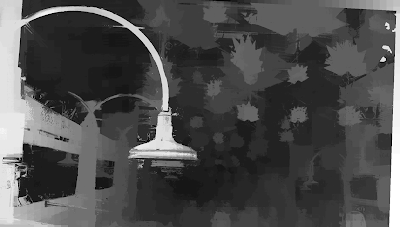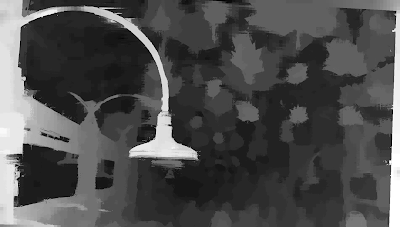## Thursday, September 28, 2017

### Case Study - DMAG5b+DMAG9b

The main parameter to tinker with when using DMAG5b is the radius. The lower the radius, the more noise. The larger the radius, the smoother the depth map is gonna be but the more inaccurate at object boundaries.

Let's stick with that depth map (for no particular reason) and post-process it with DMAG9b. The main parameters to tinker with in DMAG9b are the two sample rates (space and range aka color) and lambda (the smoothing multiplier).Depth map obtained by DMAG5b using radius = 16 and post-processed by DMAG9b using sample_rate_spatial = 32, sample_rate_range = 8, and lambda = 0.25.

What happens if you decrease the spatial sample rate from 32 to 16?Depth map obtained by DMAG5b using radius = 16 and post-processed by DMAG9b using sample_rate_spatial = 16, sample_rate_range = 8, and lambda = 0.25.

I think it's a bit better. I tried decreasing the range (color) sample rate from 8 to 4 but there was no improvement.

What happens if you increase lambda from 0.25 to 1.0?Depth map obtained by DMAG5b using radius = 16 and post-processed by DMAG9b using sample_rate_spatial = 16, sample_rate_range = 8, and lambda = 1.0.

Maybe it's a bit better although debatable. I tried to go the other way and decrease lambda but there was no improvement. So, let's call it a day and stop here.